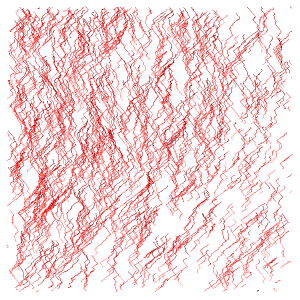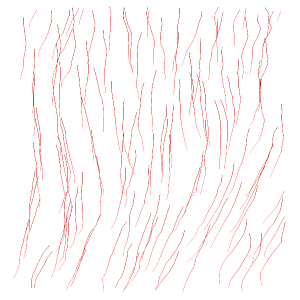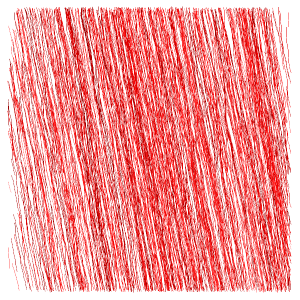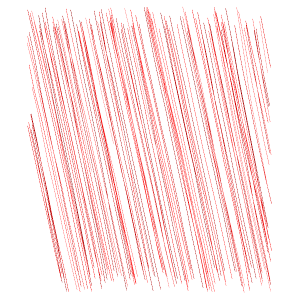## Streamlines (ImageXd)

Streamline allows the tracking of channels or structures from a vector field (for example, from Diffusion). It creates streamlines based on seed points and a vector field.

You can found a Matlab implementation here:
Kupczik K, Stark H, Mundry R, Neininger F, Heidlauf T, Röhrle O (2015). Reconstruction of muscle fascicle architecture from iodine-enhanced microCT images: a combined texture mapping and streamline approach. Journal of Theoretical Biology 382 (7): 34–43. DOI:10.1016/j.jtbi.2015.06.034 / ISSN:0022-5193

In this example, we use a part of an image stack from Julian Sartori (Thanks for the support!), which shows the achilles tendon of a rat.

And the results from Diffusion.

First step
Calculate the streamlines from the vector field.

▸ ImageXd

``` vector.load "stack_vector.nii.gz" vector.smooth 3 //vector.stream // calculate streamlines with steps 1 for all seed points vector.stream.random 1000 line.save "stream.lines" // output for cloud2 line.save.trackvis "stream.trk" // output for the software Trackvis vector.load "stack_reduce.nii.gz" line.new //vector.stream // calculate streamlines with steps 1 for all seed points vector.stream.random 1000 line.save "stream_reduce.lines" // output for cloud2 line.save.trackvis "stream_reduce.trk" // output for the software Trackvis ```

▸ More details``` // vector.stream.seeds 1 // 0:all, 1(default):only at new points, 2:only at new points around -1..1, ... // vector.stream.snakes 0 // 0(default):all, 1:only for new points, 2:only for new points around -1..1, ... // vector.stream.steps 1 // streamlines with steps 1(default) (i.e. 0.5 or 2) // vector.stream.angles 0 // streamlines with segment angle 0°(default) (i.e. 5°, 10°) // vector.stream.stop.angle 180 // streamlines stops for wrong directions > 180°(default) (i.e. > 45°) // vector.stream.stop.length 0 // streamlines stops for too long streamlines (0(default)=diagonal of bounding box) // vector.stream.nodes // calculate stream lines like vector.stream and increments corresponding scalar value // Note: Streamlines are stored in the respective coordinate system (offset) with the specified resolution (scale)! // vector.stream // calculate streamlines for all seed points from a vector field // vector.stream.random 100 // calculate 100 streamlines // vector.stream.mask mask 1 <2..10> ... // calculate streamlines for all masked seed points from a vector field // vector.stream.mask.random 100 mask 1 <2..10> ... // calculate 100 streamlines for masked seed points from a vector field // vector.stream.muscle // calculate streamlines (only if length left = length right +/- steps) for seed points from a vector field // vector.stream.muscle.random 100 // calculate 100 streamlines (only if length left = length right +/- steps) for seed points from a vector field // Note: A zero point <0,0,0> calculates the center point of your stack! // vector.stream.slice <10,10,10> <1,1,0> // calculate streamlines for all points on a plane (point*normal) from a vector field // vector.stream.slicex 10 // calculate streamlines for all points on the x plane (value*<1,0,0>) from a vector field // vector.stream.slicey 10 // calculate streamlines for all points on the y plane (value*<0,1,0>) from a vector field // vector.stream.slicez 10 // calculate streamlines for all points on the z plane (value*<0,0,1>) from a vector field ```

Second step
Evaluation (with cloud2 please use *.lines).stream.lines (x,y view)stream_reduce.lines (x,y view)stream.lines (y,z view)stream_reduce.lines (y,z view)

▸ Cloud2

``` calculate.fit.lines.degree 5 1 ```stack.lines (x,y view)stack.lines (y,z view)